Courses

# The Direct Stiffness Method: Beams - 2 GATE Notes | EduRev

## GATE : The Direct Stiffness Method: Beams - 2 GATE Notes | EduRev

The document The Direct Stiffness Method: Beams - 2 GATE Notes | EduRev is a part of the GATE Course Structural Analysis.
All you need of GATE at this link: GATE

Beam (global) Stiffness Matrix.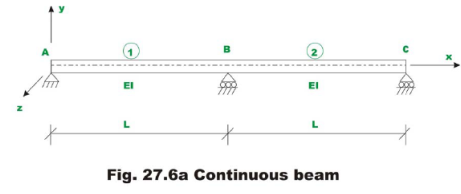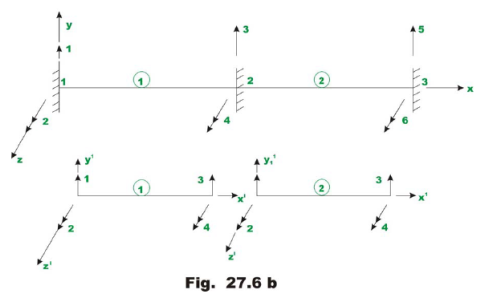For the case of continuous beam, the x - and x'- axes are collinear and other axes (y and y', z and z') are parallel to each other. Hence it is not required to transform member stiffness matrix from local co-ordinate system to global coordinate system as done in the case of trusses. For obtaining the global stiffness matrix, first assume that all joints are restrained. The node and member numbering for the possible degrees of freedom are shown in Fig 27.6b. The continuous beam is divided into two beam members. For this member there are six possible degrees of freedom. Also in the figure, each beam member with its displacement degrees of freedom (in local co ordinate system) is also shown. Since the continuous beam has the same moment of inertia and span, the member stiffness matrix of element 1 and 2 are the same. They are,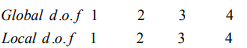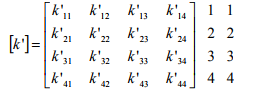(27.6a)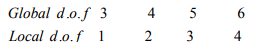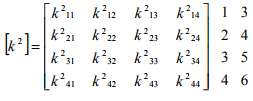(27.6b)

The local and the global degrees of freedom are also indicated on the top and side of the element stiffness matrix. This will help us to place the elements of the element stiffness matrix at the appropriate locations of the global stiffness matrix. The continuous beam has six degrees of freedom and hence the stiffness matrix is of the order 6 x 6.  Let [K] denotes the continuous beam stiffness matrix of order 6 x 6. From Fig. 27.6b, [K] may be written as,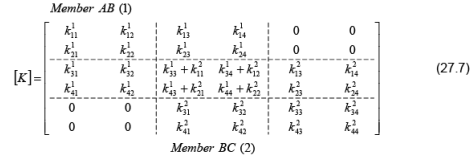The 4 x 4 upper left hand section receives contribution from member 1 (AB) and 4 x 4 lower right hand section of global stiffness matrix receives contribution from member 2. The element of the global stiffness matrix corresponding to global degrees of freedom 3 and 4 [overlapping portion of equation (27.7)] receives element from both members 1 and 2.

Consider a continuous beam ABC as shown in Fig. 27.7.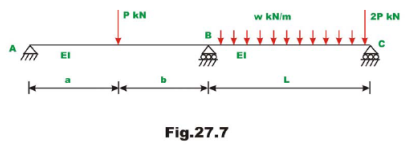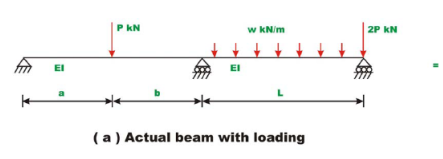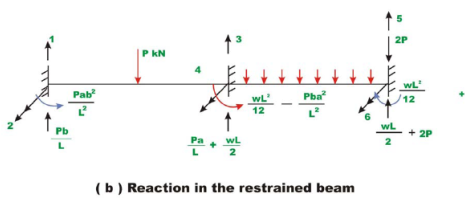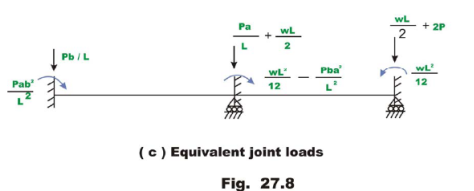The loading on the beam shown in Fig. 27.8(a), is equal to the sum of Fig. 27.8(b) and Fig. 27.8(c). In Fig. 27.8(c), the joints are restrained against displacements and fixed end forces are calculated. In Fig. 27.8(c) these fixed end actions are shown in reverse direction on the actual beam without any load. Since the beam in Fig. 27.8(b) is restrained (fixed) against any displacement, the displacements produced by the joint loads in Fig. 27.8(c) must be equal to the displacement produced by the actual beam in Fig. 27.8(a). Thus the loads shown in Fig. 27.8(c) are the equivalent joint loads .Let p1,p2,p3,p4,p5 and p6 be the equivalent joint loads acting on the continuous beam along displacement degrees of freedom 1,2,3,4,5 and 6 respectively as shown in Fig. 27.8(b). Thus the global load vector is,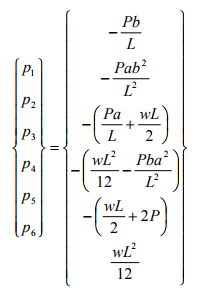(27.8)

Solution of equilibrium equations

After establishing the global stiffness matrix and load vector of the beam, the load displacement relationship for the beam can be written as,

{P} = [K]{u}                          (27.9)

where {p} is the global load vector, {u} is displacement vector and [K] is the global stiffness matrix. This equation is solved exactly in the similar manner as discussed in the lesson 24. In the above equation some joint displacements are known from support conditions. The above equation may be written as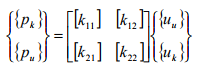(27.10)

where {pk} and {uk} denote respectively vector of known forces and known displacements. And {pu}, {uu} denote respectively vector of unknown forces and unknown displacements respectively. Now expanding equation (27.10),

{pk} = [k11]{uu} + [k12]{uk}                                     (27.11a)

{pu} = [k21]{uu} + [k22]{uk}                                    (27.11b)

Since {uk} is known, from equation 27.11(a), the unknown joint displacements can be evaluated. And support reactions are evaluated from equation (27.11b), after evaluating unknown displacement vector.

Let R1, R3 and R5 be the reactions along the constrained degrees of freedom as shown in Fig. 27.9a. Since equivalent joint loads are directly applied at the supports, they also need to be considered while calculating the actual reactions. Thus,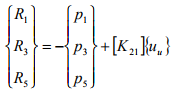(27.12)

The reactions may be calculated as follows. The reactions of the beam shown in Fig. 27.9a are equal to the sum of reactions shown in Fig. 27.9b, Fig. 27.9c and Fig. 27.9d.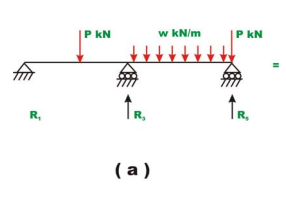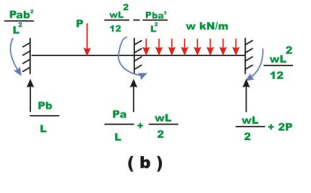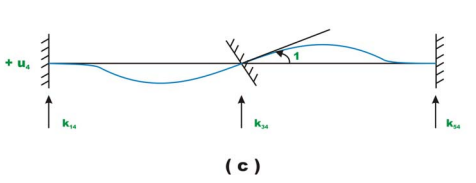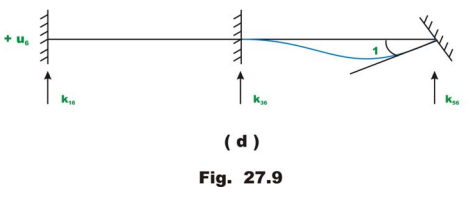From the method of superposition,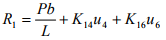(27.13a)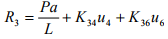(27.13b)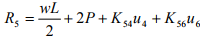(27.13c)

or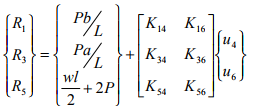(27.14a)

Equation (27.14a) may be written as,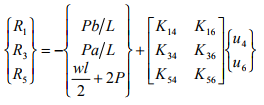(27.14b)

Member end actions q1,q2,q3,q4 are calculated as follows. For example consider the first element 1.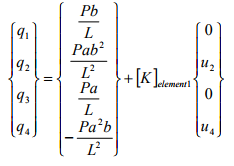(27.16)

In the next lesson few problems are solved to illustrate the method so far discussed.

Summary

In this lesson the beam element stiffness matrix is derived from fundamentals. Assembling member stiffness matrices, the global stiffness matrix is generated. The global load vector is defined. The global load-displacemet relation is written for the complete beam structure. The procedure to impose boundary conditions on the load-displacement relation is discussed. With this background, one could analyse continuous beam by the direct stiffness method.

Offer running on EduRev: Apply code STAYHOME200 to get INR 200 off on our premium plan EduRev Infinity!

## Structural Analysis

30 videos|122 docs|28 tests

,

,

,

,

,

,

,

,

,

,

,

,

,

,

,

,

,

,

,

,

,

;